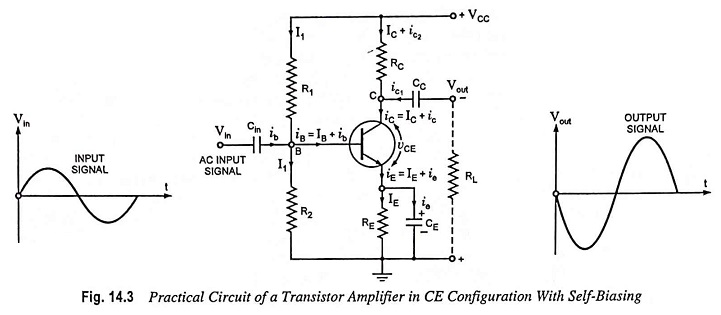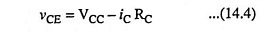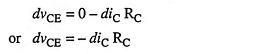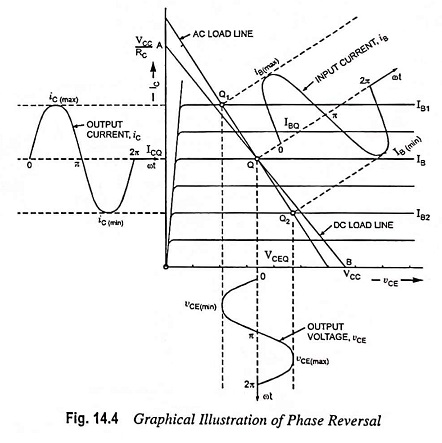## Phase Reversal in Single Stage Transistor Amplifier:

In a common emitter configuration, the output voltage increases in the negative direction when the input signal increases in the positive direction and vice versa. This is called Phase Reversal in Single Stage Transistor Amplifier and causes a phase difference of 180° between the input signal voltage Vin and output voltage Vout.Consider a CE amplifier circuit shown in Fig. 14.3, the signal being fed at the input terminals (i.e., between base and emitter) and the output is taken from the collector and emitter ends.

The instantaneous output voltage vCE is given asReactance of coupling capacitor CC is negligible at ordinary signal frequencies and so it may be considered a short for the output signal.

With the increase in the signal voltage in the positive half cycle, the base current increases causing increase in collector current and so the voltage drop iCRC. As VCC is constant, therefore, output voltage vCE decreases. Thus we can say that as the signal voltage increases in positive direction, the output voltage increases in the negative direction i.e., there is a phase difference of 180° between output and input. Conversely on negative half cycle of the input signal voltage, lesser current flows through collector resistance RC and so voltage drop across it decreases. For this reason output voltage vCE increases.

The above phenomenon can be proved mathematically as below :

Differentiating Eq. (14.4), we haveThe negative sign indicates that the output voltage is 180° out of phase with the input signal voltage.The above fact i.e., Phase Reversal in Single Stage Transistor Amplifier in CE configuration can be illustrated graphically with the aid of output characteristics and ac load line, as shown in Fig. 14.4. The base current varies between IB1 and IB2 with IB as the zero signal base current. From figure we see that when the base current is maximum in the positive direction, vCE becomes maximum in the negative direction and vice versa. Thus, the input and output voltages are in phase opposition i.e., the transistor has produced a phase reversal of output voltage w.r.t. the input signal voltage.

The noteworthy points are that common base and common collector amplifiers do not produce reversal, and input and output currents are in phase in all the three configurations.

Scroll to Top